## ML Aggarwal Class 10 Solutions for ICSE Maths Chapter 20 Heights and Distances Ex 20

These Solutions are part of ML Aggarwal Class 10 Solutions for ICSE Maths. Here we have given ML Aggarwal Class 10 Solutions for ICSE Maths Chapter 20 Heights and Distances Ex 20

More Exercises

Question 1.
An electric pole is 10 metres high. If its shadow is 10√3 metres in length, find the elevation of the sun.
Solution:
Let AB be the pole and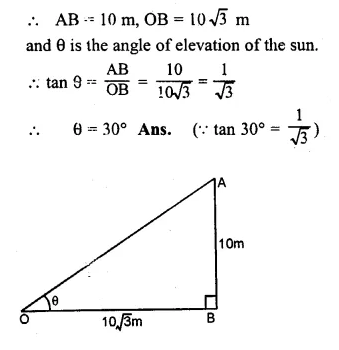Question 2.
The angle of elevation of the top of a tower from a point on the ground and at a distance of 150 m from its foot is 30°. Find the height of the tower correct to one place of decimal
Solution:
Let BC be the tower and
A is the point on the ground such that
∠A= 30° and AC = 150 m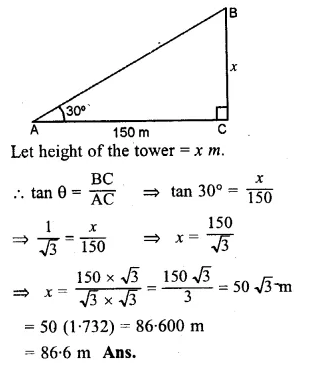Question 3.
A ladder is placed against a wall such that it just reaches the top of the wall. The foot of the ladder is 1.5 metres away from the wall and the ladder is inclined at an angle of 60° with the ground. Find the height of the wall.
Solution:
Let AB be the wall and AC be the ladder
whose foot C is 1.5 m away from B
Let AB = x m and angle of inclination is 60°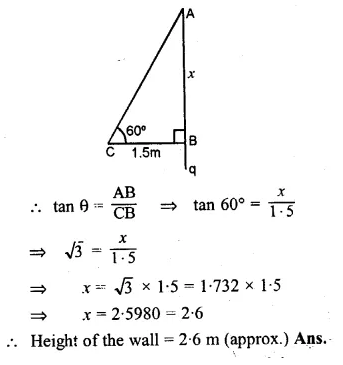Question 4.
What is the angle of elevation of the sun when the length of the shadow of a vertical pole is equal to its height.
Solution:
Let AB be the pole and CB be its shadow
and θ is the angle of elevation of the sun.
Let AB = x m, then BC = x m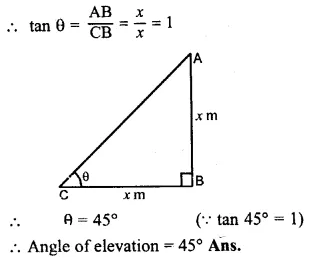Question 5.
A river is 60 m wide. A tree of unknown height is on one bank. The angle of elevation of the top of the tree from the point exactly opposite to the foot of the tree on the other bank is 30°. Find the height of the tree.
Solution:
Let AB be the tree and BC is the width of the river
and C is the point exactly opposite to B on the other bank
and angle of elevation is 30°.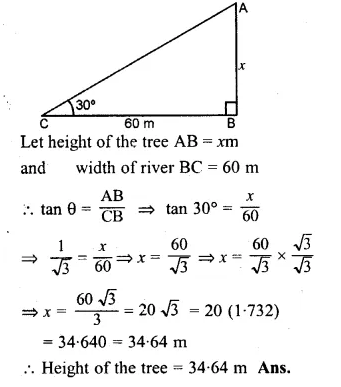Question 6.
From a point P on level ground, the angle of elevation of the top of a tower is 30°. If the tower is 100 m high, how far is P from the foot of the tower ?
Solution:
Let AB be the tower and P is at a distance of x m from B, the foot of the tower.
While the height of the tower AB = 100 m
and angle of elevation = 30°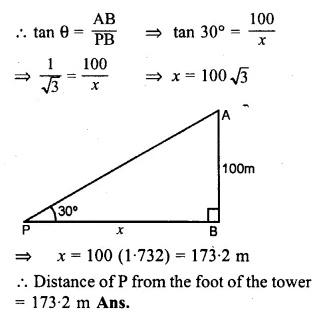Question 7.
From the top of a cliff 92 m high, the angle of depression of a buoy is 20°. Calculate to the nearest metre, the distance of the buoy from the foot of the cliff. (2005)
Solution:
Let AB be cliff whose height is 92 m
and C is buoy making depression angle of 20°.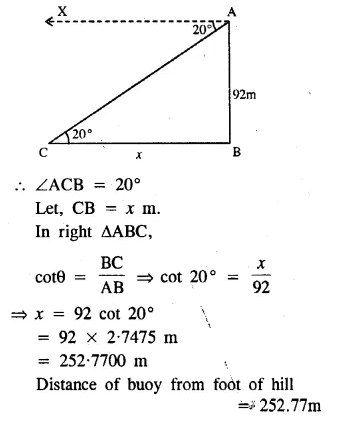Question 8.
A boy is flying a kite with a string of length 100 m. If the string is tight and the angle of elevation of the kite is 26°32′, find the height of the kite correct to one decimal place, (ignore the height of the boy).
Solution:
Let AB be the height of the kite A and AC is the string
and angle of elevation of the kite is 26°32′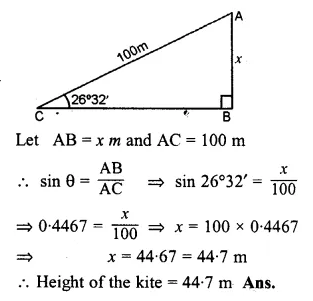Question 9.
An electric pole is 10 m high A steel wire tied to the top of the pole is affixed at a point on the ground to keep the pole upright. If the wire makes an angle of 45° with the horizontal through the foot of the pole, find the length of the wire.
Solution:
Let AB be the pole and AC be the wire
which makes an angle of 45° with the ground.
Height of the pole AB = 10 m
and let the length of wire AC = x m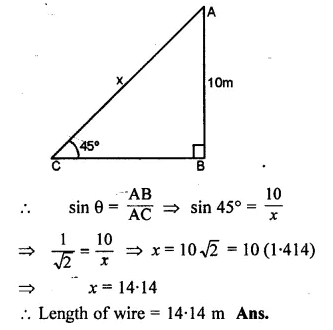Question 10.
A bridge across a river makes an angle of 45° with the river bank. If the length of the bridge across the river is 200 metres, what is the breadth of the river.Solution:
Let AB be the width of river = xm
Length of the bridge AC = 200 m
and angle with the river bank = 45°
sin θ = $$\\ \frac { AB }{ AC }$$
⇒ sin 45° = $$\\ \frac { x }{ 200 }$$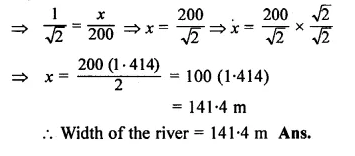Question 11.
A vertical tower is 20 m high. A man standing at some distance from the tower knows that the cosine of the angle of elevation of the top of the tower is 0.53. How far is he standing from the foot of the tower ? (2001)
Solution:
Let AB be the tower and
let a man C stands at a distance from the foot of the tower = x m
and cos θ = 0.53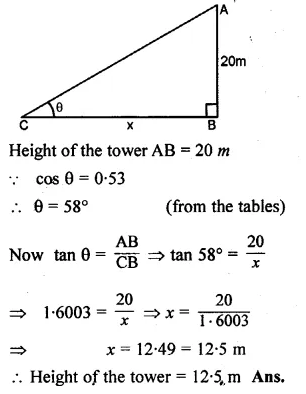Question 12.
The upper part of a tree broken by wind, falls to the ground without being detached. The top of the broken part touches the ground at an angle of 38°30′ at a point 6 m from the foot of the tree. Calculate.
(i) the height at which the tree is broken.
(ii) the original height of the tree correct to two decimal places.
Solution:
Let TR be the total height of the tree
and TP is the broken part which touches the ground
at the distance of 6 m from the foot of the tree
making an angle of 38°30′ with the ground.
Let PR = x and TR = x + y
PQ = PT = y
In right ∆PQR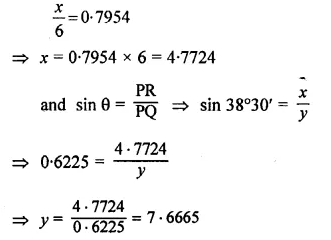Height of the tree = 4.7724 + 7.6665 = 12.4389 = 12.44 m
and height of the tree at which it is broken = 4.77 m

Question 13.
An observer 1.5 m tall is 20.5 metres away from a tower 22 metres high. Determine the angle of elevation of the top of the tower from the eye of the observer.
Solution:
In the figure, AB is tower and CD is an observer.
θ is the angle of observation from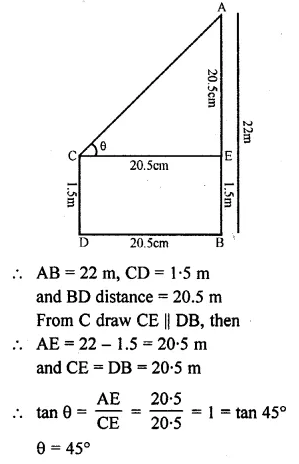Question 14.
From a boat 300 metres away from a vertical cliff, the angles of elevation of the top and the foot of a vertical concrete pillar at the edge of the cliff are 55°40′ and 54°20′ respectively. Find the height of the pillar correct to the nearest metre.Solution:
Let CB be the cliff and AC be the pillar
and D be the boat which is 300 m away from
the foot of the cliff i.e. BD = 300 m.
Angles of elevation of the top and foot of the pillar
are 55°40′ and 54°20′ respectively.
Let CB = x and AC = y
In right ∆CBD,Question 15.
From a point P on the ground, the angle of elevation of the top of a 10 m tall building and a helicopter hovering over the top of the building are 30° and 60° respectively. Find the height of the helicopter above the ground.
Solution:
let AB be the building and H is the helicopter hovering over it.
P is a point on the ground,
the angle of elevation of the top of building and helicopter are 30° and 60°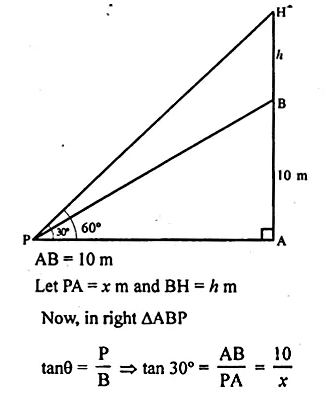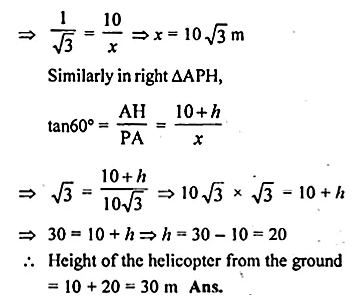Question 16.
An aeroplane when flying at a height of 3125 m from the ground passes vertically below another plane at an instant when the angles of elevation of the two planes from the same point on the ground are 30° and 60° respectively. Find the distance between the two planes at the instant.
Solution:
Let the distance between the two planes = h m
Given that, AD = 3125 m and ∠ACB = 60° and ∠ACD = 30°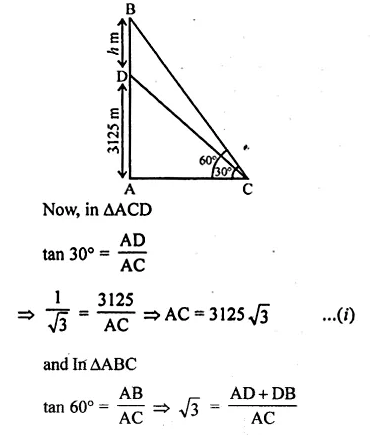Question 17.
A person standing on the bank of a river observes that the angle subtended by a tree on the opposite bank is 60° ; when he retires 20 m from the bank, he finds the angle to be 30°. Find the height of the tree and the breadth of the river. .
Solution:
Let TR be the tree and PR be the width of the river.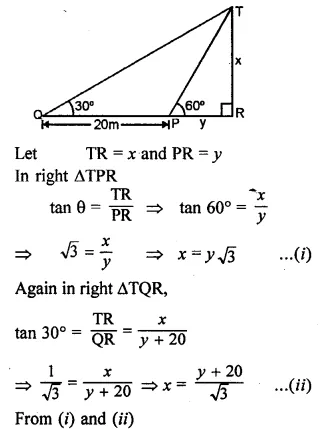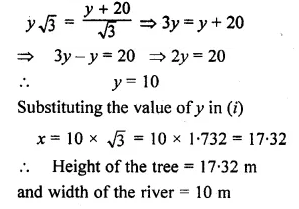Question 18.
The shadow of a vertical tower on a level ground increases by 10 m when the altitude of the sun changes from 45° to 30°. Find the height of the tower, correct to two decimal places. (2006)
Solution:
In the figure, AB is the tower,
BD and BC are the shadow of the tower in two situations.
Let BD = x m and AB = h m
In ∆ABD,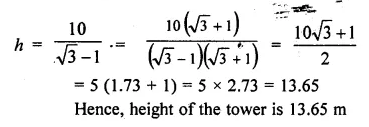Question 19.
From the top of a hill, the angles of depression of two consecutive kilometer stones, due east are found to be 30° and 45° respectively. Find the distance of two stones from the foot of the hill.
Solution:
Let A and B be the position of two consecutive kilometre stones.
Then AB = 1 km = 1000m
Let the dIstance BC = x m
∴ Distance AC = (1000 + x) mQuestion 20.
A man observes the angles of elevation of the top of a building to be 30°. He walks towards it in a horizontal line through its base. On covering 60 m the angle of elevation changes to 60°. Find the height of the building correct to the nearest me he.
Solution:
Given that
AB is a building CD = 60 m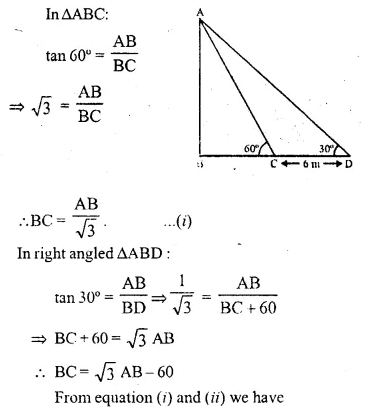Question 21.
At a point on level ground, the angle,of elevation of a vertical lower is found to be such that its tangent is $$\\ \frac { 5 }{ 12 }$$. On walking 192 m towards the tower,the tangent of the angle is found to be $$\\ \frac { 3 }{ 4 }$$. Find the height of the tower. (1990)
Solution:
Let TR be the tower and P is the point on the
ground such that tan θ = $$\\ \frac { 5 }{ 12 }$$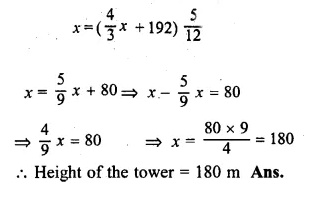Question 22.
In the figure, not drawn to scale, TF is a tower. The elevation of T from A is x° where tan x = $$\\ \frac { 2 }{ 5 }$$ and AF = 200 m. The elevation of T from B, where AB = 80 m, is y°. Calculate :
(i) The height of the tower TF.
(ii) The angle y, correct to the nearest degree. (1997)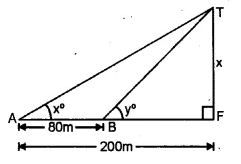Solution:
Let height of the tower TF = x
tan x = $$\\ \frac { 2 }{ 5 }$$, AF = 200 m, AB = 80 m
(i) In right ∆ATF,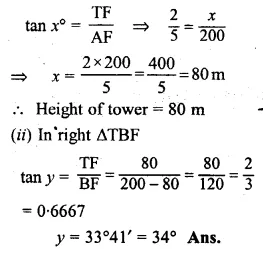Question 23.
From the top of a church spire 96 m high, the angles of depression of two vehicles on a road, at the same level as the base of the spire and on the same side of it are x° and y°, where tan x° = $$\\ \frac { 1 }{ 4 }$$ and tan y° = $$\\ \frac { 1 }{ 7 }$$. Calculate the distance between the vehicles. (1994)
Solution:
Height of the church CH.
Let A and B are two vehicles which make the angle of depression
from C are x° and y° respectively.Question 24.
In the adjoining figure, not drawn to the scale, AB is a tower and two objects C and D are located on the ground, on the same side of AB. When observed from the top A of the tower, their angles of depression are 45° and 60°. Find the distance between the two objects. If the height of the tower is 300 m. Give your answer to the nearest metre. (1998)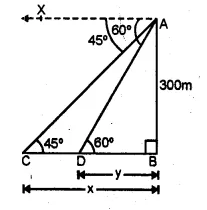Solution:
Let CB = x and
DB = y
AB = 300 m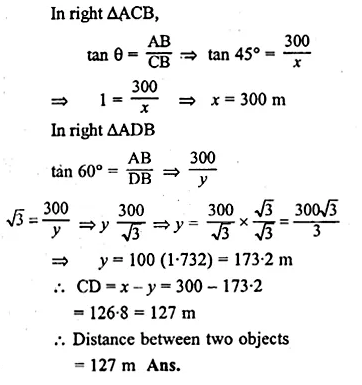Question 25.
The horizontal distance between two towers is 140 m. The angle of elevation of the top of the first tower when seen from the top of the second tower is 30°. If the height of the second tower is 60 m, find the height of the first tower.
Solution:
Let the height of first tower TR = x
height of second tower PQ = 60 m
Distance between the two towers QR = 140 m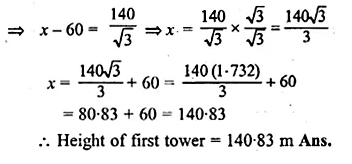Question 26.
As observed from the top of a 80 m tall lighthouse, the angles of depression of two ships on the same side of the ,lighthouse in horizontal line with its base are 30° and 40° respectively. Find the distance between the two ships. Give your answer correct to the nearest metre.
Solution:
Let AB be the lighthouse and C and D be the two ships.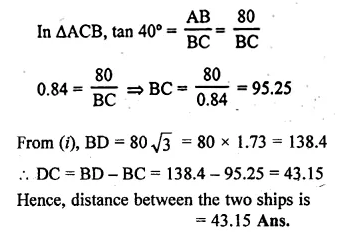Question 27.
The angle of elevation of a pillar from a point A on the ground is 45° and from a point B diametrically opposite to A and on the other side of the pillar is 60°. Find the height of the pillar, given that the distance between A and B is 15 m.
Solution:
Let CD be the pillar and let CD = x
Angles of elevation of points A and B are 45° and 60° respectively.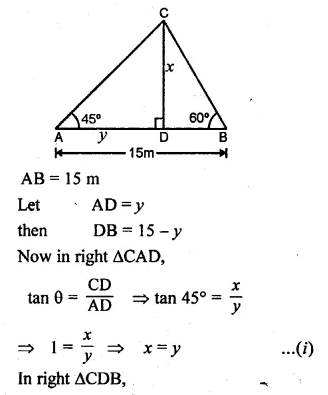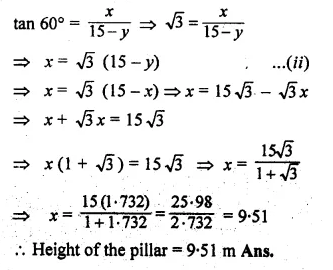Question 28.
From two points A and B on the same side of a building, the angles of elevation of the top of the building are 30° and 60° respectively. If the height of the building is 10 m, find the distance between A and B correct to two decimal places
Solution:
In ∆DBC, tan 60° = $$\\ \frac { 10 }{ BC }$$
⇒ √3= $$\\ \frac { 10 }{ BC }$$
⇒ BC = $$\frac { 10 }{ \sqrt { 3 } }$$
∆DBC ,tan 30° = $$\\ \frac { 10 }{ BC+AB }$$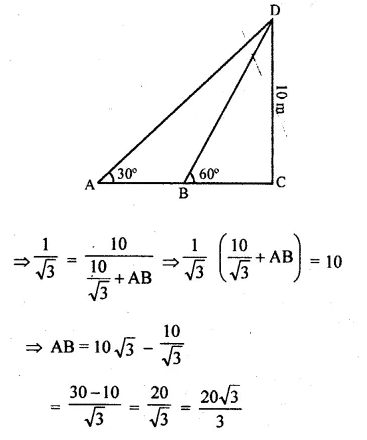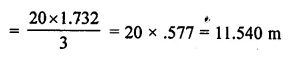Question 29.
(i) The angles of depression of two ships A and B as observed from the top of a light house 60 m high are 60° and 45° respectively. If the; two ships are on the opposite sides of the light house, find the distance between the two ships. Give your answer correct to the nearest whole number. (2017)
(ii) An aeroplane at an altitude of 250 m observes the angle of depression of two boats on the opposite banks of a river to be 45° and 60° respectively. Find the width of the river. Write the answer correct to the nearest whole number. (2014)
Solution:
(i) Let AD be the height of the lighthouse CD = 60 m
Let AD = x m, BD = y m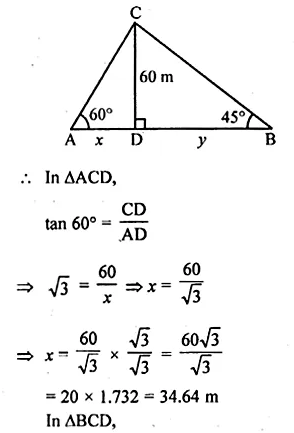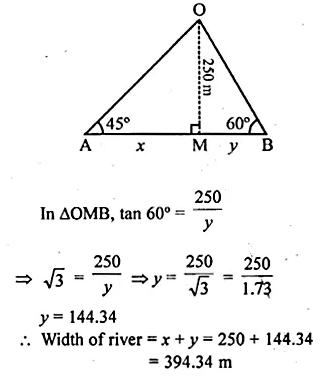Question 30.
From a tower 126 m high, the angles of depression of two rocks which are in a horizontal line through the base of the tower are 16° and 12°20′ Find the distance between the rocks if they are on
(i) the same side of the tower
(ii) the opposite sides of the tower.
Solution:
Let CD be the tower and CD = 126 m
Let A and B be the two rocks on the same line
and angles of depression are 16° and 12°20′ respectively,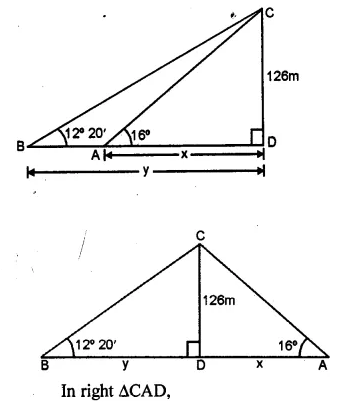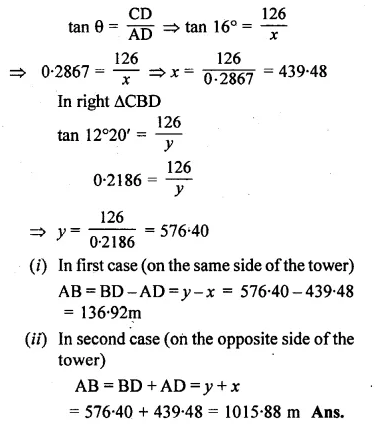Question 31.
A man 1.8 m high stands at a distance of 3.6 m from a lamp post and casts a shadow of 5.4 m on the ground. Find the height of the lamp post.
Solution:
AB is the lamp post CD is the height of man.
BD is the distance of man from the foot of the lamp
and FD is the shadow of man.
CE || DB.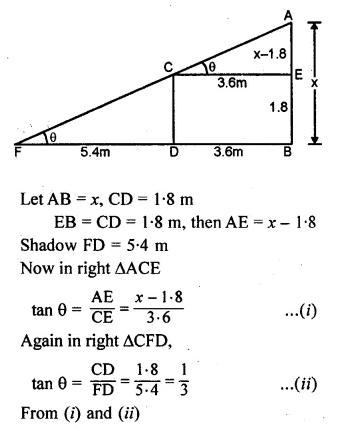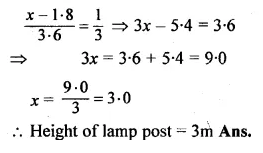Question 32.
The angles of depression of the top and the bottom of an 8 m tall building from the top of a multi-storeyed building are 30° and 45° respectively. Find the height of tire multi-storeyed building and the distance between the two buildings, correct to two decimal places.
Solution:
Let AB be the CD be the building
The angles of depression in from A, to C
and D are 30° and 45° respectively
∠ACE = 30° and ∠ADB = 45°
CD = 8 m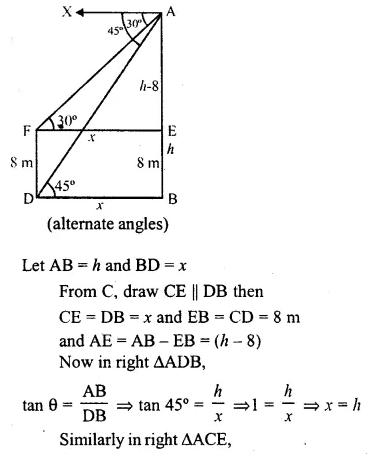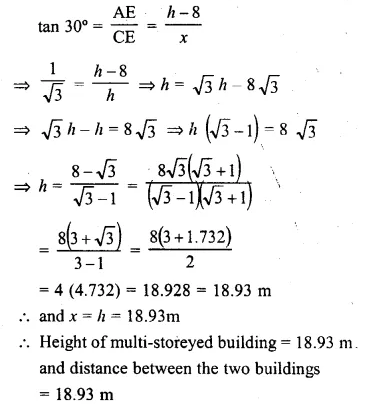Question 33.
A pole of height 5 m is fixed on the top of a tower. The angle of elevation of the top of the pole as observed from a point A on the ground is 60° and the angle of depression of the point A from the top of the tower is 45°. Find the height of the tower. (Take √3 = 1.732).
Solution:
Let QR be the tower and PQ be the pole on it
Angle of elevation from P to a point A is ∠PAR = 60°
and angle of depression from Q to A = 45°
∠QAR = 45° (alternate angle)
PQ = 5 m,Question 34.
A vertical pole and a vertical tower are on the same level ground. From the top of the pole the angle of elevation of the top of the tower is 60° and the angle of depression of the foot of the tower is 30°. Find the height of the tower if the height of the pole is 20 m.
Solution:
Let TR is tower and
PL is the pole on the same level, ground PL = 20m
From P, draw PQ || LR
then ∠ TPQ = 60° and ∠ QPR = 30°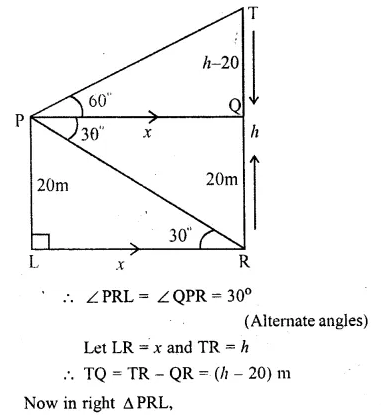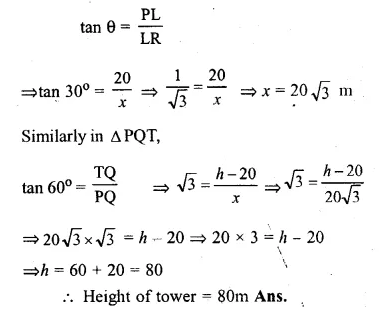Question 35.
From the top of a building 20 m high, the angle of elevation of the top of a monumenti is 45° and the angle of depression of its foot is 15°. Find the height of the monument.
Solution:
Let AB be the building and AB = 20 m and
let CD be the monument and let CD = x
The distance between the building and the monument be y,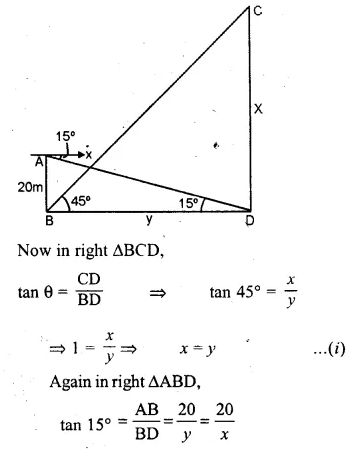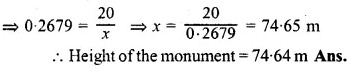Question 36.
The angle of elevation of the top of an unfinished tower at a point distant 120 m from its base is 45°. How much higher must the tower be raised so that its angle of elevation at the same point may be 60°?
Solution:
Let AB be the unfinished tower and AB = 120 m
and angle of elevation = 45°
Let x be higher raised so that
the angle of elevation becomes 60°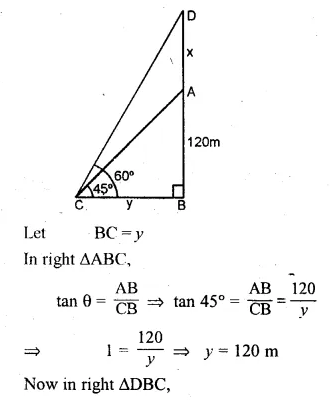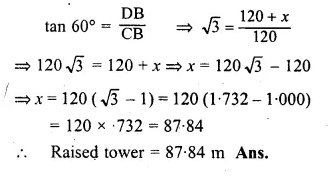Question 37.
In the adjoining figure, the shadow of a vertical tower on the level ground increases by 10 m, when the altitude of the sun changes from 45° to 30°. Find the height of the tower and give your answer, correct to $$\\ \frac { 1 }{ 10 }$$ of a metre.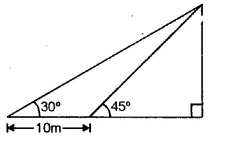[Remark. Altitude of the sun means angle of elevation of the sun.]
Solution:
Let TR be the tower and TR = h ;
Let BR = x,
AB = 10 m
Angles of elevation from the top of the tower
at A and B are 30° and 45° respectively.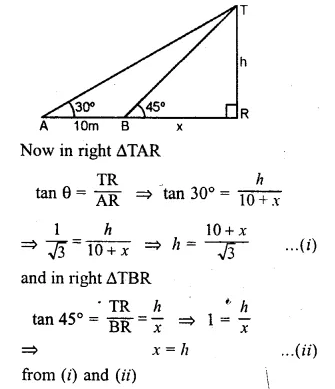Question 38.
An aircraft is flying at a constant height with a speed of 360 km/h. From a point on the ground, the angle of elevation of the aircraft at an instant was observed to be 45°. After 20 seconds, the angle of elevation was observed to be 30°. Determine the height at which the aircraft is flying (use √3 = 1.732)
Solution:
Speed of aircraft = 360 km/h
Distance covered in 20 seconds = $$\\ \frac { 360X20 }{ 60X60 }$$ = 2 km
E is the fixed point on the ground
and CD is the position of AB in height of aircraft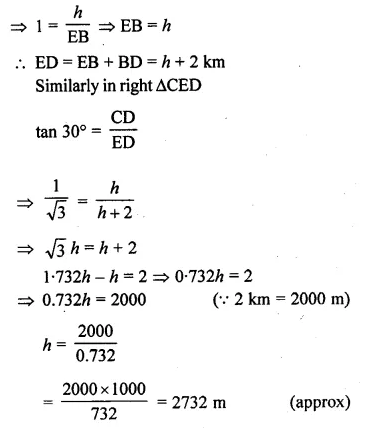We hope the ML Aggarwal Class 10 Solutions for ICSE Maths Chapter 20 Heights and Distances Ex 20 help you. If you have any query regarding ML Aggarwal Class 10 Solutions for ICSE Maths Chapter 20 Heights and Distances Ex 20, drop a comment below and we will get back to you at the earliest.